# Particle in a Box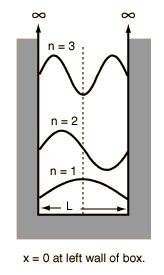Assume the potential U(x) in the time-independent Schrodinger equation to be zero inside a one-dimensional box of length L and infinite outside the box. For a particle inside the box a free particle wavefunction is appropriate, but since the probability of finding the particle outside the box is zero, the wavefunction must go to zero at the walls. This constrains the form of the solution to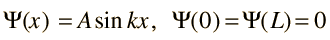which requires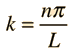... (Compare to string modes)
and after normalization, the wavefunction is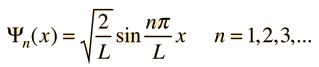Discussion of significance Quantized energies Containment energies Finite-walled box
 3-D particle in box
Index

Schrodinger equation concepts

 HyperPhysics***** Quantum Physics R Nave
Go Back

# Normalization, Particle in BoxFor the particle in a box with infinite walls, the probability must be equal to one for finding it within the box. The condition for normalization is then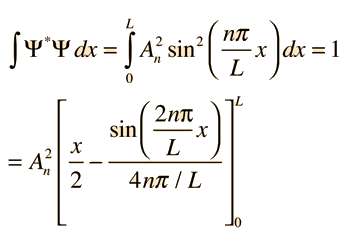The sin terms drop out, leaving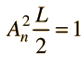... (Show form of integration)
so the normalized wavefunctions are:Index

Schrodinger equation concepts

 HyperPhysics***** Quantum Physics R Nave
Go Back

# Particle in Finite-Walled Box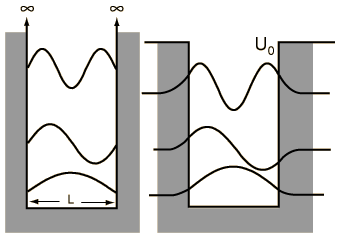For the finite potential well, the solution to the Schrodinger equation gives a wavefunction with an exponentially decaying penetration into the classicallly forbidden region. Confining a particle to a smaller space requires a larger confinement energy. Since the wavefunction penetration effectively "enlarges the box", the finite well energy levels are lower than those for the infinite well.

### Energy level example

Index

Schrodinger equation concepts

 HyperPhysics***** Quantum Physics R Nave
Go Back

# Particle in Finite-Walled Box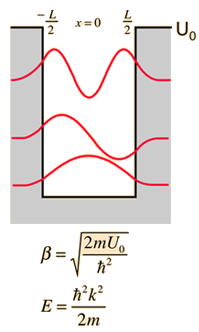For a potential which is zero over a length L and has a finite value for other values of x, the solution to the Schrodinger equation has the form of the free-particle wavefunction for -L/2 < x < L/2 and elsewhere must satisfy the equation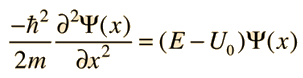With the substitution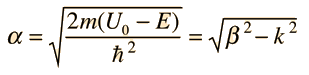this may be written in the form: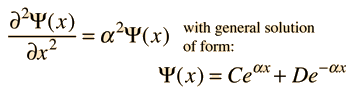### Solution from fitting boundary conditions

Index

Schrodinger equation concepts

 HyperPhysics***** Quantum Physics R Nave
Go Back

# Finite Well Energy Levels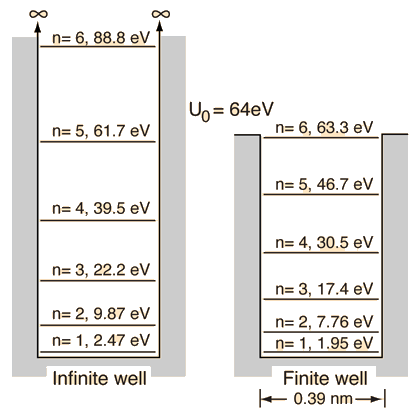The energy levels for an electron in a potential well of depth 64 eV and width 0.39 nm are shown in comparison with the energy levels of an infinite well of the same size.

### Solution from fitting boundary conditions

Index

Schrodinger equation concepts

References: Rohlf Sec 7-3
Blatt, Sec. 7-6.

 HyperPhysics***** Quantum Physics R Nave
Go Back